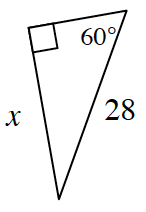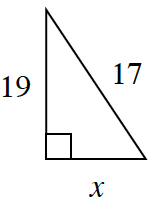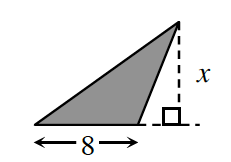### Home > INT2 > Chapter 12 > Lesson 12.2.3 > Problem12-115

12-115.

For each triangle below, determine the value of $x$, if possible. Name the triangle relationship that you used. If the triangle cannot exist, explain why.

1.$30°- 60°- 90°$ pattern

1.Why is the length of the hypotenuse significant in this particular triangle?

1.Area of the shaded region is $96$ square units.

Use the area to work backwards to find the value of $x$.